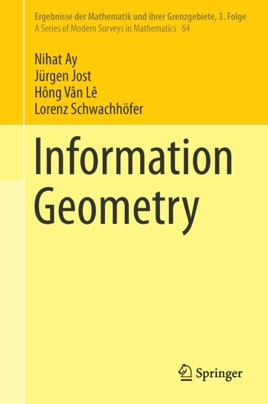• \$109.99

## Publisher Description

The book provides a comprehensive introduction and a novel mathematical foundation of the field of information geometry with complete proofs and detailed background material on measure theory, Riemannian geometry and Banach space theory.  Parametrised measure models are defined as fundamental geometric objects, which can be both finite or infinite dimensional. Based on these models, canonical tensor fields are introduced and further studied, including the Fisher metric and the Amari-Chentsov tensor, and embeddings of statistical manifolds are investigated.

This novel foundation then leads to application highlights, such as generalizations and extensions of the classical uniqueness result of Chentsov or the Cramér-Rao inequality.  Additionally, several new application fields of information geometry are highlighted, for instance hierarchical and graphical models, complexity theory, population genetics, or Markov Chain Monte Carlo.

The book will be of interest to mathematicians who are interested in geometry, information theory, or the foundations of statistics, to statisticians as well as to scientists interested in the mathematical foundations of complex systems.